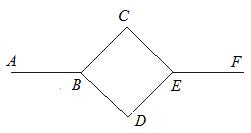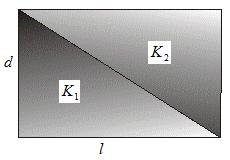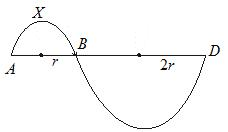# Assignment 1 on subjective type questions on heat transfer

Subjective Questions of Heat Transfer
Q-1:
A rod is composed of two section whose length are L1 and L2 and heat conductivities are K1 and K2 respectivity.One end of the rod is kept at T1 and other end is kept at T2.The rod is enclosed in a thermally insulating sheath.
a. Find the temperature of the interface.
b. Find the equivalent thermal conductivity of the system.
c. Find the heat current in the rod

Q-2:
An 1 mole of a gas whose adiabatic coefficient is 1.4 is at an initial temperature T0 ,is enclosed in a cylindrical vessel fitted with a light piston. The surrounding temperature is TS and atmospheric pressure is Pa.Heat may be conducted between the surrounding and gas through the bottom of the cylinder. The bottom has surface area A, thickness is L1 and thermal conductivity K.Asumming all the changes to be slow and (Ts > T0).
a, find the temperature of the gas as a function of the time t.
b. Find the distance moved by the gas as function of the time t.

Q-3:
A body A of heat capacity s is kept in a surrounding where temperature is Ta.It cools down according Newton laws of cooling. The mass of the body is m and the body initial temperature is T0.
a. Find the value of constant k if the temperature at time t1 is T1
b. finds the maximum heat body can lose.
c. Find the time at which body loses 80% of the total heat loss.
d. plots the graph for the logarithm of the numerical value of the temperature difference of the body A and its surrounding with respect to time t.

Q-4:
A rod of length l with thermally insulated lateral surface consists of a material whose heat conductivity coefficient varies with temperature given below
K=αT1/2 where α is constant.
the end of the rod are kept at the temperature T1 and T2 where T1 > T2.
Find the function T(x) where x is the distance from end where the temperature is T1
And the heat flow through the rod

Q-5:Six identical rods AB,BC,BD,CE,and DF are joined as shown in figure. The length ,crossectional area of each rod is A and L.
The thermal conductivities of the rods are as below
AB->K
BC and BD -> K/2
CE ,DE and EF ->K
The ends A and F are maintained at the temperature T1 and T2.Asumming no loss of heat to the atmosphere, find
a. Thermal resistance of the six rods
b. Thermal resistance of the whole system
c. The temperature at the points TB,TC,TD,TE
d. The heat flow ratio among the path BCE and BDE.

Q-6:
A spherical shell has inner and outer radii as a and b respectively. And temperature at the inner and outer surface area are Ta and Tb And Ta > Tb.K is the thermal conductivity of the spherical shell.
Find the heat current through the shell

Q-7:A rod of length l as shown is figure above with thermally insulated lateral surface is made of two material whose thermal conductivies are K1 and K2 respectively. The crossectinal area is a square having edge d. Temperature at the ends are T1 and T2(T1 > T2)
a, Find the heat flow
b. Equivalent thermal conductivity of the Rod

Q-8:

A cubical Block of mass 1 kg and edge 5 cm is heated to 227 °C.It is kept in a chamber which is maintained at the temperature 27 °C. Assuming the cubical block is having value of e=.5
a. Find the amount of radiation falling on the block.
b. Find the net amount of heat flow outside the block.
c. Find the rate at which temperature decrease at the beginning if specific heat=400J/kg-K
d. if the chamber is maintained at the same temperature as the block. Find the heat absorbed and heat radiated by the block.

σ=6.0x10-8 W/m2-K4

Q-9:
One end of the rod of length L is inserted in furnace which is maintained at temperature T1.The sides of the rod are covered with insulating material. Other end emits radiation with emissivity e. The temperature of the surrounding Ts.Let T2 is the temperature of the end in steady state.
a. Find the thermal conductivity of the Rod
b. Find the heat flow in the Rod.

Q-10:

A Rod is initially at a uniform temperature at T1.One end is kept at T1 and other end is kept in a furnace maintained at temperature at T2.(T2 >T1).The Surface of the rod is insulated so that heat can flow lengthwise along the rod.Lenght of the Rod is L, area A and thermal conductivity of the Rod is K.Consider a short cylindrical element of the rod of unit lenght.If the temperature gradient at the one end of the element is K'.Find the rate of flow across the element.

Q-11:
The tungsten element of the electric lamp has as surface area A and Power is P and emissivity is .4
a. Find the temperature of the filament
b. if the tungsten filament behave like blackbody ,find the % increase in power required to maintain the same temperature.

Q-12:
A sphere, a cube and a circular plate have the same mass and are made of same material. All of them are at the same temperature T.Which one will have maximum rate of cooling.

Q-13:
A hole of radius R1 is made centrally in a circular disc of thickness d and radius R2.The inner surface is maintained at temperature T1 and other surface is maintained at T2 (T1 > T2).Thermal conductivity of the circular plate is K.
a. Find the temperature as a function of radius from center
b. Find the heat flow per unit time

Q-14:
Two metallic sphere X & Y are made of same material and have identical surface finish. Let m1,m2 are the masses of the spheres X & Y respectively. Both the sphere is at the same temperature. Both the sphere is placed in the same room having lower temperature. But they are thermally insulated from each other.
Also (m1/m2)=n. If the initial rate of cooling of sphere X to Y is (1/4)1/3.Find the value of n.

Q-15:A system is shown in figure.
It is made of semicircular Rod (AXB) which is joined at its ends to a straight rod AB of same material and the same crossection .The straight Rod form a diameter of the other rod. Similarly semicircular Rod (BYD) which is joined at its ends to a straight rod of same material and cross-section area.
a. Find the ratio of heat transfer across AXB & AB
b. Find the ratio of heat transfer across BYD & BD

Solutions